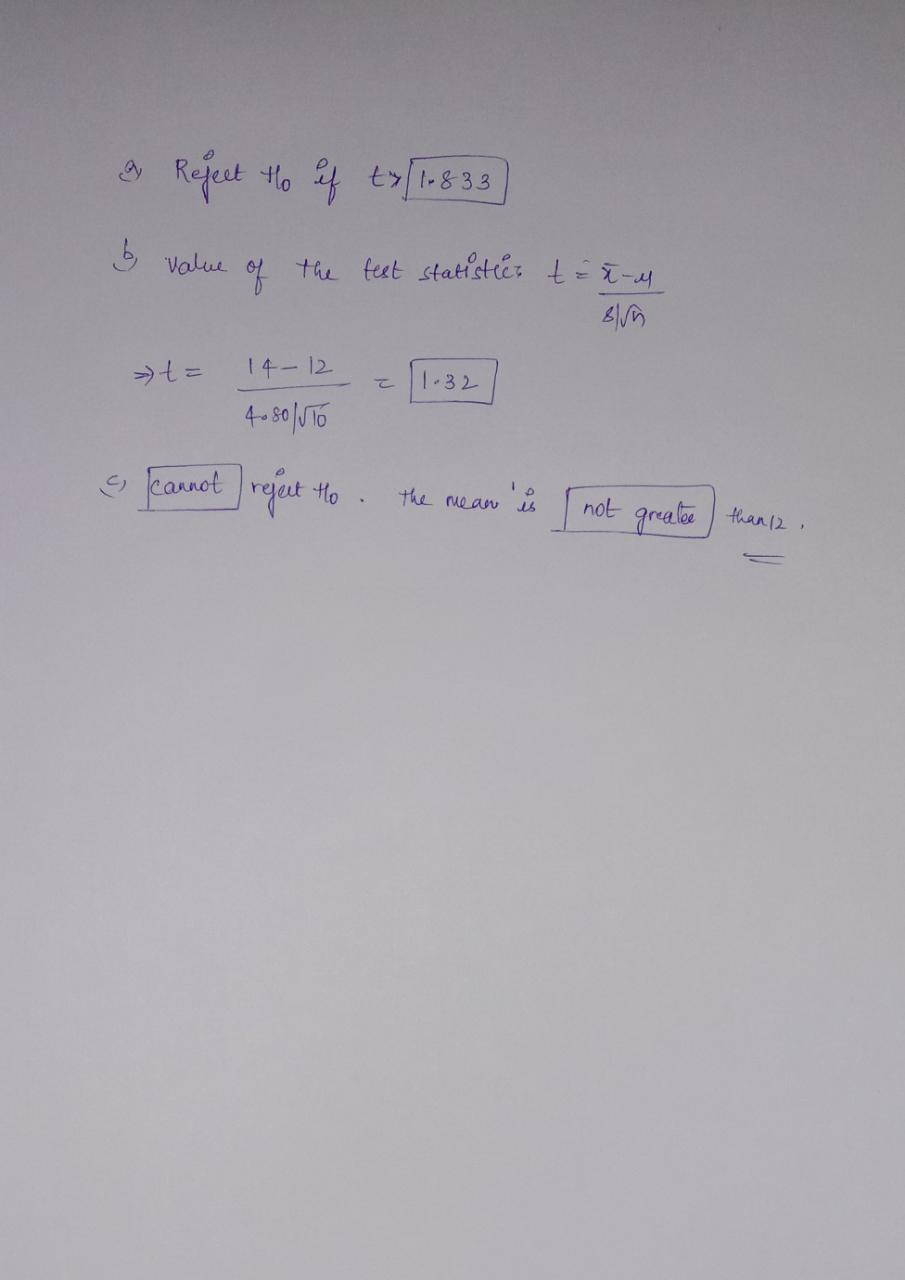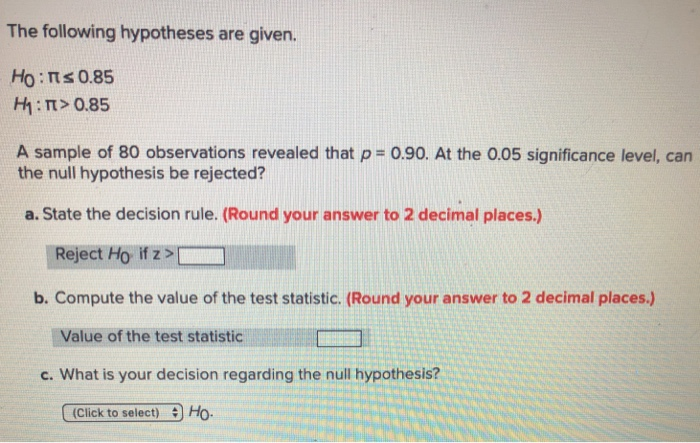Question

# Given the following hypothesis:      H0 : μ ≤ 12 H1 : μ > 12 For...

 Given the following hypothesis: H0 : μ ≤ 12 H1 : μ > 12
 For a random sample of 10 observations, the sample mean was 14 and the sample standard deviation 4.80. Using the .05 significance level:
 (a) State the decision rule. (Round your answer to 3 decimal places.)
 (Click to select)Cannot rejectReject H0 if t >
 (b) Compute the value of the test statistic. (Round your answer to 2 decimal places.)
 Value of the test statistic
 (c) What is your decision regarding the null hypothesis?
 (Click to select)RejectCannot reject H0. The mean (Click to select)isis not greater than 12.#### Earn Coins

Coins can be redeemed for fabulous gifts.

Similar Homework Help Questions
• ### The following hypotheses are given. H0 : π ≤ 0.83 H1 : π > 0.83 A...

The following hypotheses are given. H0 : π ≤ 0.83 H1 : π > 0.83 A sample of 100 observations revealed that p = 0.87. At the 0.10 significance level, can the null hypothesis be rejected? State the decision rule. (Round your answer to 2 decimal places.) Compute the value of the test statistic. (Round your answer to 2 decimal places.) What is your decision regarding the null hypothesis? Do not reject H0. Reject H0. question 2: The number of...

• ### Given the following hypotheses: H0: μ ≤ 13 H1: μ > 13 A random sample of...

Given the following hypotheses: H0: μ ≤ 13 H1: μ > 13 A random sample of 10 observations is selected from a normal population. The sample mean was 11 and the sample standard deviation 3.6. Using the 0.05 significance level: State the decision rule. (Round your answer to 3 decimal places.) Compute the value of the test statistic. (Negative answers should be indicated by a minus sign. Round your answer to 3 decimal places.) What is your decision regarding the...

• ### Given the following hypotheses: H0: μ = 600 H1: μ ≠ 600 A random sample of...

Given the following hypotheses: H0: μ = 600 H1: μ ≠ 600 A random sample of 16 observations is selected from a normal population. The sample mean was 609 and the sample standard deviation 6. Using the 0.10 significance level: State the decision rule. (Negative amount should be indicated by a minus sign. Round your answers to 3 decimal places.) Reject H0 when the test statistic is outside the interval ( , ). ? Compute the value of the test...

• ### The null and alternate hypotheses are:    H0 : μ1 = μ2 H1 : μ1 ≠...

The null and alternate hypotheses are:    H0 : μ1 = μ2 H1 : μ1 ≠ μ2    A random sample of 11 observations from Population 1 revealed a sample mean of 21 and sample deviation of 3.5. A random sample of 7 observations from Population 2 revealed a sample mean of 23 and sample standard deviation of 3.8. The underlying population standard deviations are unknown but are assumed to be equal. At the .05 significance level, is there a...

• ### The following information is available. H0: μ = 48 H1: μ ≠ 48 The sample mean...

The following information is available. H0: μ = 48 H1: μ ≠ 48 The sample mean is 47, and the sample size is 38. The population standard deviation is 7. Use the .05 significance level. 1. value: 2.00 points Required information Is this a one- or two-tailed test? One-tailed test Two-tailed test 2. value: 2.00 points Required information What is the decision rule? Reject H0 if z < -1.96 or z > 1.96 Reject H0 if -1.96 < z <...

• ### Consider the following hypothesis test. H0: μ ≤ 12 Ha: μ > 12 A sample of...

Consider the following hypothesis test. H0: μ ≤ 12 Ha: μ > 12 A sample of 25 provided a sample mean x = 14 and a sample standard deviation s = 4.28. (a) Compute the value of the test statistic. (Round your answer to three decimal places.) (b) Use the t distribution table to compute a range for the p-value. p-value > 0.2000.100 < p-value < 0.200    0.050 < p-value < 0.1000.025 < p-value < 0.0500.010 < p-value < 0.025p-value <...

• ### A sample of 37 observations is selected from a normal population. The sample mean is 29,...

A sample of 37 observations is selected from a normal population. The sample mean is 29, and the population standard deviation is 5. Conduct the following test of hypothesis using the 0.05 significance level.    H0 : μ ≤ 26 H1 : μ > 26 a. Is this a one- or two-tailed test? "One-tailed"-the alternate hypothesis is greater than direction. "Two-tailed"-the alternate hypothesis is different from direction. b. What is the decision rule? (Round your answer to 3 decimal places.)...

• ### The null and alternate hypotheses are:    H0 : μd ≤ 0 H1 : μd >...

The null and alternate hypotheses are:    H0 : μd ≤ 0 H1 : μd > 0    The following sample information shows the number of defective units produced on the day shift and the afternoon shift for a sample of four days last month.    Day       1 2 3 4   Day shift 12     12     16    19      Afternoon shift 10     10     12    15    At the .05 significance level, can we conclude there are more...

• ### A sample of 31 observations is selected from a normal population. The sample mean is 11, and the population standard deviation is 3. Conduct the following test of hypothesis using the 0.05 significanc...

A sample of 31 observations is selected from a normal population. The sample mean is 11, and the population standard deviation is 3. Conduct the following test of hypothesis using the 0.05 significance level. H0: μ ≤ 10 H1: μ > 10 Is this a one- or two-tailed test? One-tailed test Two-tailed test What is the decision rule? Reject H0 when z > 1.645 Reject H0 when z ≤ 1.645 What is the value of the test statistic? (Round your...

• ### The following hypotheses are given. НО : Ms 0.85 H1 : π > 0.85 A sample...The following hypotheses are given. НО : Ms 0.85 H1 : π > 0.85 A sample of 80 observations revealed that p- 0.90. At the 0.05 significance level, can the null hypothesis be rejected? a. State the decision rule. (Round your answer to 2 decimal places.) Reject Ho if z b. Compute the value of the test statistic. (Round your answer to 2 decimal places.) Value of the test statistic c. What is your decision regarding the null hypothesis? (Click...